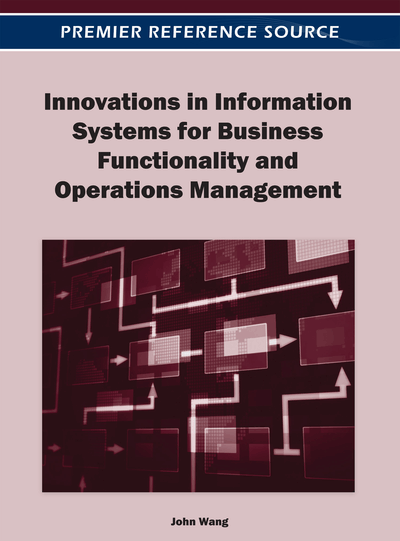# Numerical Studies on Reformulation Techniques for Continuous Network Design with Asymmetric User Equilibria

Xuegang (Jeff) Ban (Xuegang (Jeff) BanRensselaer Polytechnic Institute, USA), Michael Ferris (University of Wisconsin-Madison, USA) and Henry X. Liu (University of Minnesota, USA)
Copyright: © 2012 |Pages: 20
DOI: 10.4018/978-1-4666-0933-4.ch008
OnDemand PDF Download:
Available
\$37.50
No Current Special Offers

## Abstract

In this article, we aim to find the most effective reformulation techniques to solve the MPCC (mathematical program with complementarity constraints) model that we proposed recently for continuous network design problems under asymmetric user equilibria. The MPCC model is based on a link-node nonlinear complementarity formulation for asymmetric user equilibria. By applying various reformulation techniques for the lower level nonlinear complementarity, the original bilevel formulation can be converted to a single level nonlinear programming problem. We show that certain reformulations are more effective than others to solve the proposed MPCC model. Recommendations are thus provided on how to choose a reformulation of the continuous network design problem that can be solved effectively and/or efficiently.
Chapter Preview
Top

## Introduction And Motivation

The continuous network design problem (CNDP) has been proposed and studied for the last several decades, aiming to determine the optimal capacity expansion for a set of selected links in a given transportation network (see Morlok et al. 1973; Abdulaal & LeBlanc, 1979; Tan, Gershwin, & Athana, 1979; Marcotte, 1983; Suwansirikul, Friesz, & Tobin, 1987; Friesz, Cho, Mehta, Tobin, & Anandalingam, 1992; Yang, 1997; Josefsson & Patriksson, 2008). CNDP can be formulated as a bilevel programming problem—the upper level is a nonlinear programming problem (NLP) to minimize certain system cost, and the lower level is a user equilibrium (UE) problem to account for drivers’ route choice behavior. Such a bilevel formulation is also termed mathematical programs with equilibrium constraints (MPEC), which are generally non-convex and nonsmooth, and even fail to satisfy certain constraint qualifications (e.g., the Mangasarian Fromovitz Constraint Qualification, see Luo, Pang, & Ralph, 1996). Therefore, solving the bilevel CNDP problem is usually challenging, and despite the extensive research so far, no algorithm has been developed that can efficiently solve CNDPs with guaranteed convergence (Yang & Bell, 2001).

One of the widely used solution techniques for CNDP is the sensitivity-based algorithm (Tobin & Friesz, 1988), based on the observation that although CNDP is not smooth everywhere, it is relatively smooth (Friesz, Cho, Mehta, Tobin, & Anandalingam, 1992), that is, smooth almost everywhere. Therefore, the gradient of the objective function with respect to the upper level decision variable can be calculated using the sensitivity analysis technique. Such a method however may fail especially when the problem is not smooth at the optimal solution (Josefsson & Patriksson, 2008). More recent approaches for modeling CNDP focus on reformulating the problem using certain forms of smooth gap functions for the lower-level UE. Meng, Yang, and Bell (2001) formulated CNDP as a bilevel problem with the lower level UE an NLP. Using a particular gap function for the lower level problem, the bilevel model was converted to a single level (yet smooth) NLP and solved using the augmented Lagrangian method. Nevertheless, the model was based on the symmetry assumption on the lower-level UE problem. Here we note that a UE is symmetric if the travel time of any link depends on only the traffic flow of itself (also called separable) or the dependence of travel times on link flows are symmetric; otherwise, an asymmetric UE (AUE) will have to be considered if the dependence is not symmetric. For formal definitions of symmetric and asymmetric UEs, one can refer to Patriksson (1994, equation 2.44). Symmetric UE is therefore a special case of the asymmetric UE (AUE), and can be formulated as an NLP (Beckmann, McGuire, & Winsten, 1956). Beckmann-type NLP formulation however does not exist for AUE, which has to be formulated as a nonlinear complementarity problem (NCP) or variational inequality (VI). By defining certain gap functions for the lower level VI, Marcotte and Zu (1996) transferred the bilevel problem to a single level one and solved using a penalty method. Patriksson and Rockafellar (2002) presented a reformulation technique to convert a CNDP into a constrained and locally Lipschitz minimization problem which can be further solved using an algorithm proposed in the same paper. However, neither Marcotte and Zu (1996) nor Patriksson and Rockafellar (2002) tested their models using well-known CNDP examples in the transportation field.

## Complete Chapter List

Search this Book:
Reset